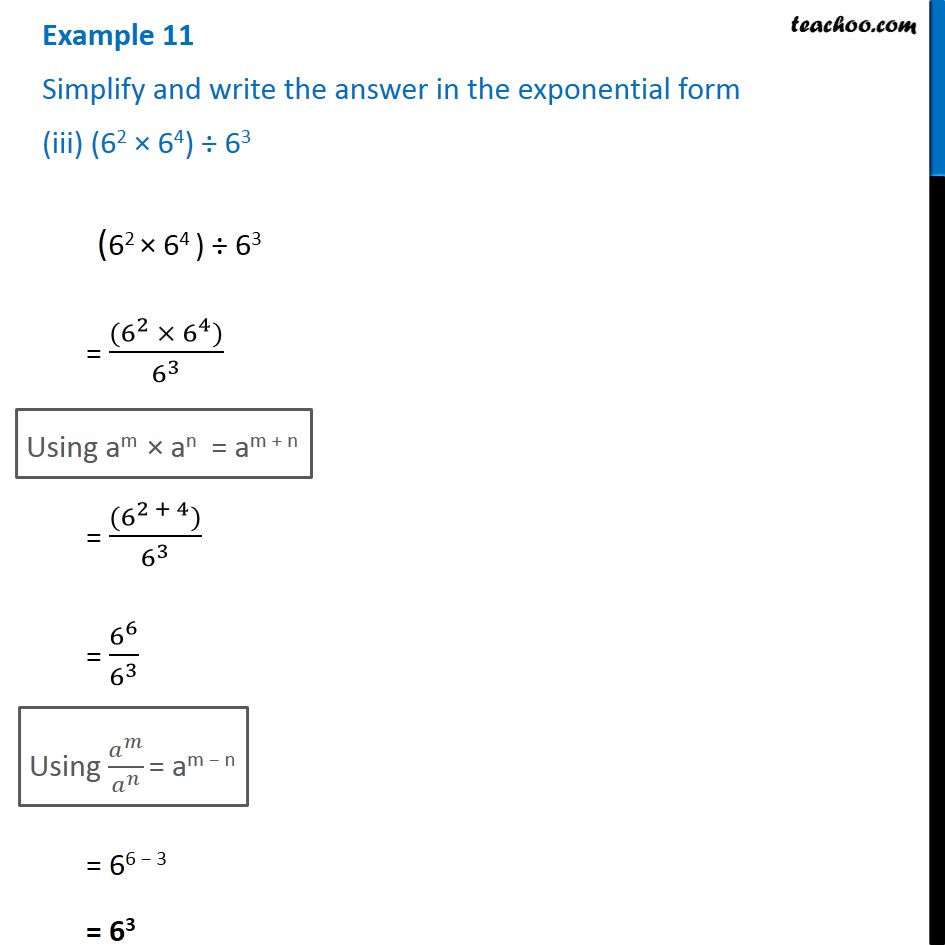1. Chapter 13 Class 7 Exponents and Powers
2. Serial order wise
3. Examples

Transcript

Example 11 Simplify and write the answer in the exponential form (iii) (62 × 64) ÷ 63 (62 × 64 ) ÷ 63 = ((6^2 ×〖 6〗^4))/6^3 Using am × an = am + n = ((6^(2 + 4)))/6^3 = 6^6/6^3 Using 𝑎^𝑚/𝑎^𝑛 = am − n = 66 − 3 = 63